# Faraday's Second Law of Electrolysis↪ This law deals about the relationship between mass of substance deposited on or liberated from an electrode and their equivalent weight.

↪ It states that, "When the same quantity of electricity is passed through different electrolytes connected in series; the amount of substance deposited on or liberated from the respective electrodes are proportional to their chemical equivalents or equivalent weight."

i.e. m  E which is the mass of substance deposited.
or, m = KE
K = m/E where E = Equivalent weight and K = Proportionality constant

⁕ ﻿Experimental Verification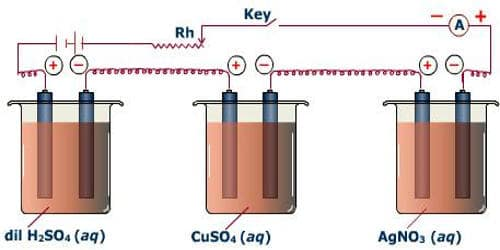fig: Experimental Verification of Faraday's second law of electrolysis

Let the same quantity of electricity is passed through different voltameter connected in series containing aqueous solution of H2SO4, CuSO4, AgNO3, etc. as shown in figure.

The amount of substance deposited or liberated from cathode of these electrolytic cell on passing one coulomb of electricity is equal to the electrochemical equivalent.

Therefore, according to Faraday's Second Law,

For First Cell,
mass of hydrogen deposited ∝ equivalent weight of hydrogen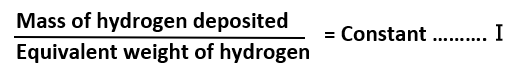For Second Cell,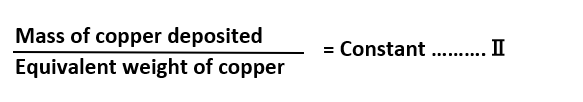For Third Cell,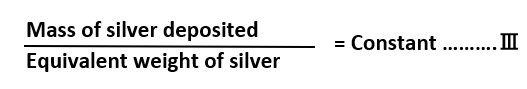From equations Ⅰ, Ⅱ and Ⅲ,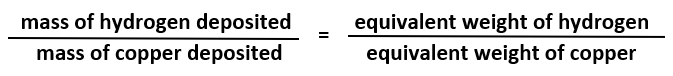or,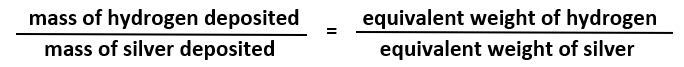or,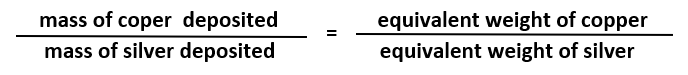⁕ ﻿Significance of the Faraday's Law

i. It is applicable to calculate the charge carried by single ions.
ii. To calculate equivalent weight of metals.